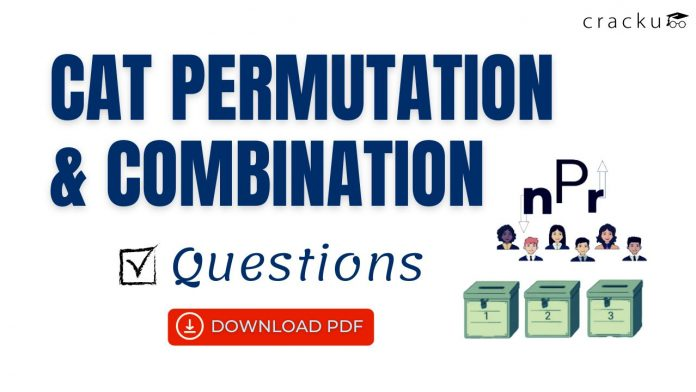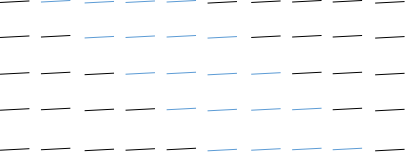0
64Permutation and Combination is one of the key topics in the CAT Quantitative Ability (QA) Section. If you’re not very strong in this topic, it is essential that you know the basics of the CAT Permutation and Combination. Also, do check out all the Permutation and Combination questions from the CAT Previous Papers with detailed video solutions. This article will look into some important P&C questions for the CAT Exam. If you want to practice these important Permutation and Combination questions for CAT, you can download the PDF, which is completely Free.

Question 1: A ten-digit telephone number must have all 10 digits from 0 to 9, and the left-most digit can be 0. What is the total number of possible telephone numbers that can be issued if it is known that all the prime digits(2, 3, 5, 7) come together and no prime digit occupies the place at either end of the number?

a) 4! x 6!

b) 7! x 4!

c) 5! x 6!

d) 5 x 5! x 6!

Solution:

Approach 1:

The 10 digits are represented in the following figure. The 4 consecutive prime digits are represented in blue.There is no other possible way to represent the prime digits. Now, in each case, the prime digits can arrange themselves in 4! way and the other digits can arrange in 6! ways.

Hence, 5 x 4! x 6! = 5! x 6!.

Approach 2:

Let us calculate the number of cases where primes are together.

The number of cases = 7! x 4!

Now we need to subtract only those cases, where they are together and also one of them is at any end.

Hence, we subtract 2 x 4! x 6!

We get the answer as 7! x 4! – 2 x 4! x 6!  = 4! ( 7! – 2 x 6! ) = 4! ( 6! x 7 – 6! x 2) = 4! x 6! x 5 = 5! x 6!.

Question 2: What is the dictionary ranking of MBRELLAU among all the words made by shuffling the alphabets of the word UMBRELLA?

a) 13218

b) 12318

c) 18312

d) 15672

e) 12381

Solution:

A – 7!/2! = 2520
B – 7!/2! = 2520
E – 7!/2! = 2520
L – 7! = 5040
MA – 6!/2! = 360
MBA – 5!/2! = 60
MBE – 5!/2! = 60
MBL – 5! = 120
MBRA – 4!/2! = 12
MBREA – 3!/2! = 3
MBRELA – 2! = 2
MBRELLAU – 1
Sum = 13218

Question 3:  A fair die is rolled successively 4 times .Find the Probability that in each turn the number that appears on the die is greater than the number which appeared on the die in the previous turn.

a) $\frac{10}{6^4}$

b) $\frac{5}{432}$

c) $\frac{1}{6^4}$

d) $\frac{5}{216}$

e) None of the above

Solution:

The possible outcomes will be:
(1,2,3,4)
(1,2,3,5)
(1,2,3,6)
(1,2,4,5)
(1,2,4,6)
(1,2,5,6)
(1,3,4,5)
(1,3,4,6)
(1,3,5,6)
(1,4,5,6)
(2,3,4,5)
(2,3,4,6)
(2,3,5,6)
(2,4,5,6)
(3,4,5,6)
Therefore Probability=$\frac{\left(Possible\ Outcomes\right)}{Total\ Outcomes}$
=$\frac{15}{6^4}$
=$\frac{15}{1296}$
=$\frac{5}{432}$
Alternatively :
we have 6 digits (1,2,3,4,5,6)
we need any 4 so we can chose 4 numbers in $^6C_4$ ways
Now, there will be only 1 way in which the numbers will be in ascending order
so probability becomes :$\frac{^6C_4}{6^4}$=$\frac{5}{432}$

Question 4: The letters of the word ‘BREAK’ are arranged in all possible ways, without repetition. The list is sorted in alphabetical order. What is
the rank of the word ‘BREAK’.

a) 46

b) 52

c) 51

d) 44

e) 45

Solution:

BREAK has 5 alphabets
B,R,E,A,K
Now we will start with the words whose 1st alphabet is A
now total words with A as starting alphabet – 4!=24
Now B with first so we will have A as second
Therefore number of words: 3!=6
BE will also have 6 words
BK will also have 6 words
Now we get BR
BRA will have 2 words
now we will have
BREAK will be fist word
Therefore rank of BREAK will be 24+18+2+1=45
Therefore option E is the correct answer.

Question 5: 100 balls are labelled with distinct numbers among 1 to 100 in a container. If Ramesh was told about this and asked to guess the probability of picking a prime numbered ball from the lot and Ramesh answers it wrong because he misheard  100 as 140 and calculates the probability accordingly. What is the difference between actual probability and the probability guessed by Ramesh ?

a) 1/280

b) 3/70

c) 1/140

d) 3/280

e) 1/70

Solution:

There are a total of 25 prime numbers below hundred.

Based on this the original probability of picking a prime numbered ball must be 25/100 = 1/4.

But since Ramesh misreads this to 140 he calculates this for 140.

Between 100 and 140  the additional prime numbers are 101, 103, 107, 109, 113, 127, 131, 137, 139.

Hence the probability that Ramesh calculates is 34/140.

The original probability is 1/4 = 35/140.

The probability calculated by Ramesh = 34/140.

The difference between the two probabilities  = 35/140 – 34/140 = 1/140

Hence, Option C is the correct choice.

Question 6: If a box contains sheets with 150 sheets with each sheet have a unique number among 1 to 150 written on top it. What is the probability that when a sheet is picked contains a number which is a multiple of 3 and of the form 7k+2 where k is a whole number ?

a) 1/30

b) 2/50

c) 7/150

d) 2/30

e) 3/50

Solution:

Among the given numbers the attribute of the chosen number must be a multiple of 3 and must be of the form 7k+2.

Since of the following attributes the numbers of form form 7k + 2 are lesser in number and hence must be counted.

The numbers less than 150 and are of the given form 7k+2 are :

2,9, 16, 23, 30, 37, 44, 51, 58, 65, 72, 79, 86, 93, 100, 107, 114, 121, 128, 135, 142, 149.

Among these numbers we need to select the numbers which are multiples of 3.

Hence we can only choose among 9, 30, 51, 72, 93, 114, 135.

Hence 7 of the 150 possibilities and the answer is 7/150

Question 7: In a throwing darts competition there must be at least 1 winner and at most 2 winners. If A, B, C are the three participants and the possibilities for winning among the different people are :
Only A wins = 1/3
Only B wins = 1/4
Both  A and B win = 1/6.
What is the probability for C becoming a winner ?

a) 1/3

b) 2/7

c) 2/5

d) 1/4

e) 1/8

Solution:

Since as per the condition mentioned in the question we can have different possibilities for winners.

Only A wins (1)  = 1/3

Only B wins    (2) = 1/4

Only C wins   (3)

Both A and B combined win (4) = 1/6

Both B and C wins combinedly  (5)

Both C and A win combinedly.  (6)

Since C must be a winner we need to to know the probability of 3, 5 and 6.

or removing the possibilities where C does not turn out to be a winner.

= 1-((1) +(2) +(4))

= $1-\ \left(\frac{1}{3}+\frac{1}{4}+\frac{1}{6}\right)$.

= 1/4

Question 8: If a box contains 45 black balls, 57 red balls, 19 green balls and 25 blue balls, find out the minimum number of balls that need to be picked up so that we definitely have one ball of each colour.

Solution:

If we need to definitely pick a ball of each colour, we need to first sort the balls according to their count.

57 red balls, 45 black balls, 25 blue balls, 19 green balls

Now, to definitely have each colour we have to count all the balls of red, black and blue and only one ball of green.

Hence, total count = 57 + 45 + 25 + 1 = 128

Question 9: Ram and Shyam are given 99 different cards numbered 1 to 99. They take turns picking out one card without replacing it till they get the card numbered 49. The one who gets the card with 49 on it is the winner. If Ram starts the game, the probability of him winning the game is $\frac{m}{n}$. where $m,n$ are co-prime numbers. What is the value of $4m-n$?

Solution:

If Ram started the game, the probability he picked out the required card in first-pick = $\frac{1}{99}$

The probability he picked out the required card in third-pick = $\frac{98}{99}\times\frac{97}{98}\times\frac{1}{97}=\frac{1}{99}$

The probability he picked out the required card in fifth-pick = $\frac{98}{99}\times\frac{97}{98}\times\frac{96}{97}\times\frac{95}{96}\times\frac{1}{95}=\frac{1}{99}$

.

.

.

.

The probability he picked out the required card in last-pick(99 pick) = $\frac{1}{99}$

Probabilty that Ram picks the card = $\frac{1}{99}+\frac{1}{99}+\frac{1}{99}….50\text{times}$ = $\frac{50}{99}$ = $\frac{m}{n}$

4m-n = 200-99 = 101

Question 10: Ram painted the six faces of a die with six different colours- Red, Yellow, White, Blue, Green and Black. What is the probability that the faces with colours- Red, Yellow and white share a common corner?

a) $\frac{1}{5}\$

b) $\frac{1}{6}\$

c) $\frac{2}{5}\$

d) $\frac{1}{3}\$

Solution:

Total number of ways of painting the 6 faces of a cube with 6 different colours-

1. We first fix one colour to one of the side.

2. The opposite side of the cube can be coloured in 5 ways.

3. Now, we colour the rest 4 colours using the circular arrangement. This can be done in 3! ways.

.’. Sample space= $5\times3!\$

For the favourable outcome,

1. Fix Red, Yellow and White to 1 corner. This will be a circular arrangement around the corner. It can be done in (3-1)! = 2 ways.

2. Once the positions of Red, Yellow and White are fixed, the remaining 3 colours can be arranged in 3! ways.

.’. Required probability= $\frac{3!\times2}{5\times3!\ }=\ \frac{2}{5}\$

Question 11: How many ordered pairs $(a,b,c,d)$ are there such that they satisfy the inequality $4 < a+b+c+d \le 20$ where a,b,c and d are whole numbers?

a) 10696

b) 10626

c) 10616

d) 10556

Solution:

$a,b,c, d \le 0$ We can split the solution in 2 sets to solve

i) Solve for $a+b+c+d \le 20$, let us add a dummy $e$ such that $e \ge0$ such that $a+b+c+d+e = 20$

The number of solutions possible = $^{20+5-1}C_{5-1}=^{24}C_4$

From this cases we have to remove the cases $a+b+c+d \le 4$ as we are given

ii) Solve for $a+b+c+d \le 4$, let us add a dummy $e$ such that $e \ge0$ such that $a+b+c+d+e = 4$

The number of solutions possible = $^{4+5-1}C_{5-1}=^{8}C_4$

Total possible cases are $^{24}C_4-^8C_4$ =10626-70 = 10556

Question 12: How many times does the digit 9 appear in total in all even numbers starting from 2 to 9999?

Solution:

If we consider all even numbers from 0 to 9999, we get (9999 + 1)/2 = 5000 such numbers.

In these 5000 numbers, the unit’s digit is always 0, 2, 4, 6 or 8.

Hence, if we consider the number to be ABCD D can not be 9.

A, B, and C can take any values from 0 to 9.

So, the total number of digits comprising A, B and C in all 5000 appearances = 5000 x 3(for A, B and C) = 15000

Now, among these 15000 digits, digits 0 to 9 appear an equal number of times.

Hence, 9 will appear 1/10 th of the times.

Hence, total number of appearances of 9 = 15000/10 = 1500.